# Qlik Sense Documents

Qlik Sense documentation and resources.

Announcements
Skip the ticket, Chat with Qlik Support instead for instant assistance.

# Creating Mini Chart in Qlik Sense tables

cancel
Showing results for
Did you mean:MVP

## Creating Mini Chart in Qlik Sense tables

Hi all,

Mini Charts have always been a good feature in QlikView and It's really 'frustrating' that this has not been added (yet) in Qlik Sense.

With hopes that the Qlik Team will think about such improvements for the next releases, let's discuss here how to create our own mini chart in a Qlik Sense Table.

The following expressions might seem 'complicated' at first sight, but remember that It will be generic.

Thus, their use will be very easy.

Let's begin :

Suppose we have:

As a dimension: OICA Region

As measures:

Sum(Sales)

Sum("Commercial Vehicle Sales")

Sum(Sales) / (Sum(Sales)+Sum("Commercial Vehicle Sales"))

Here is our current table: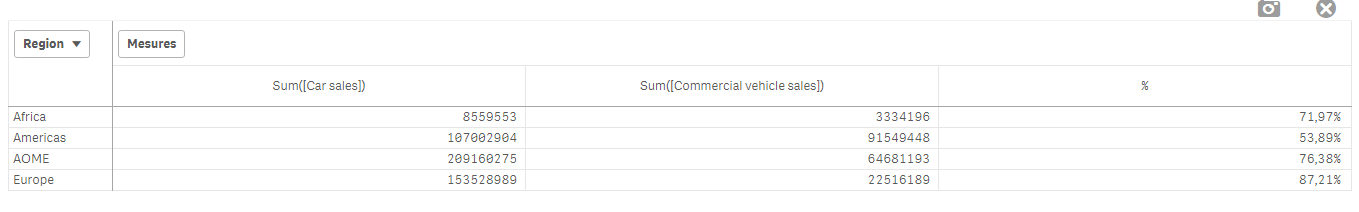Now, we want to add, a mini 'bar' to represent the %:

How to do so:

As an expression:

=repeat('█',

rangemax(rangemin( ceil(((column(1)/column(2))-1)*2.5),10),0)

)

With that, we repeat the square sign and precise the limits.

And as a text Color expression:

if( (sum([Car sales])/(sum([Car sales])+sum([Commercial vehicle sales])))>=0.8,Green(),

if((sum([Car sales])/(sum([Car sales])+sum([Commercial vehicle sales])))>=0.7,Yellow(),

Red()))

Result: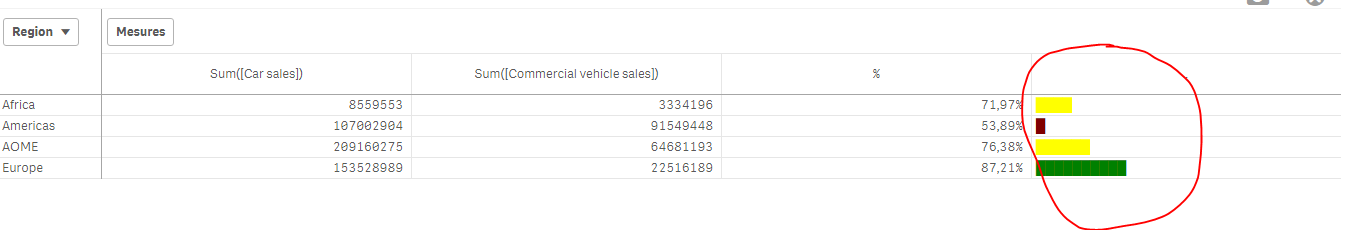We can also Add the % in front of the bar by adding it in the expression:

From :

=repeat('█',

rangemax(rangemin( ceil(((column(1)/column(2))-1)*2.5),10),0)

)

To:

=repeat('█',

rangemax(rangemin( ceil(((column(1)/column(2))-1)*2.5),10),0)

) & num((sum([Car sales])/(sum([Car sales])+sum([Commercial vehicle sales]))),'# ##0,00%')

Result:You can even alter it as follow:

repeat(chr(09608),round( sum([Car sales])/(sum([Car sales])+sum([Commercial vehicle sales])) * 10))

&repeat(chr(09617),10-round( sum([Car sales])/(sum([Car sales])+sum([Commercial vehicle sales])) *10))

&num((sum([Car sales])/(sum([Car sales])+sum([Commercial vehicle sales]))),'# ##0,00%')

&if(sum([Car sales])/(sum([Car sales])+sum([Commercial vehicle sales]))>0.7,'▲','▼')

result: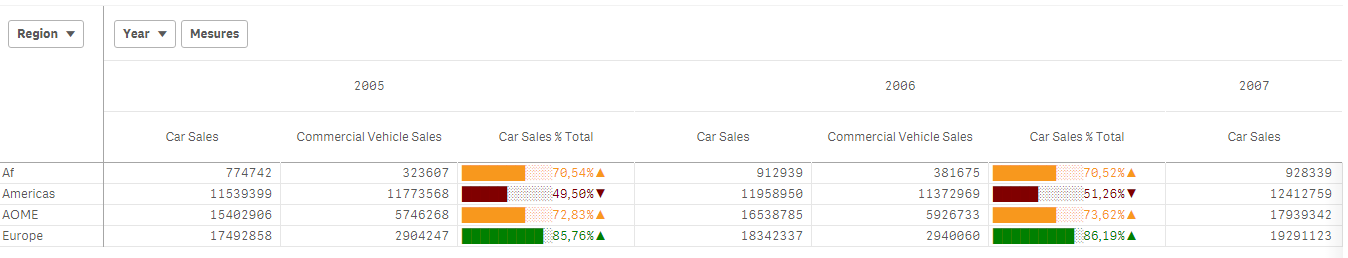Now, let's suppose we want to add another mini chart, a trend one, to see the % expression by Year:

If it has increased or decreased from a year to the next one.

How to do so?

Expression:

=concat(

aggr(

if((sum([Car sales])/(sum([Car sales])+sum([Commercial vehicle sales]))) > above((sum([Car sales])/(sum([Car sales])+sum([Commercial vehicle sales])))),

'▀',if((sum([Car sales])/(sum([Car sales])+sum([Commercial vehicle sales]))) < above((sum([Car sales])/(sum([Car sales])+sum([Commercial vehicle sales])))),'▄',' ')

)

,[OICA region], (Year,(NUMERIC, ASCENDING))

)

,'',[OICA region])

Wirh:

Red: Our Dimension

Green: The trending dimension

Blue: our expression compared to the previous one (by year, the trending expression)

If expression by Year > expression Previous Year then , Square Up

If expression by Year < expression Previous Year then , Square down

Result:Special thanks to blog Von Heldendaten in which I found this:

QlikView + Qlik Sense Blog von Heldendaten: Qlik Sense Calendar Measures & "Minicharts" in Tabellen.

Hope you like it !

Omar,

Tags (2)Master

Hi Omar

Thanks à lot for this very interesting postAnonymous
Not applicable

Cunning yet simple.  I like it.Partner - Creator

Simple and very effective - thanks for sharing this Omar.Partner - Master

Hi Omar,

Thanks for the very useful information.Partner - Creator II

Just copy and paste them: ▁▂▃▄▅▆▇█

{"▁▂▃▄▅▆▇█▇▆▅▄▃▂▁","▂▁▄▃▆▅█▇","█▇▆▅▄▃▂▁▂▃▄▅▆▇█","▁▅█▆▅▃▂▇▄▅"}

Use ORD('') to get CHR code.

Cool post. Thanks for idea.Anonymous
Not applicable

Thank you so much.... It was very helpful...MVP

Nice to have, can i expect any thing related same in Trend like Line chart ?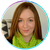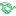Great idea! Thank a lotMVP

I got it, By help of extension Qlik Branch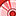Creator

Hi,

Can someone give me a simple example of using mini chart in qlik sense?

I don't succeed apply the example from this page. My result is the same graphic image on all the column of table.

Thank you,

Magdalena

Version history
Last update:
‎2017-03-28 05:27 AM
Updated by: